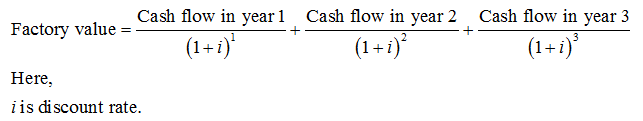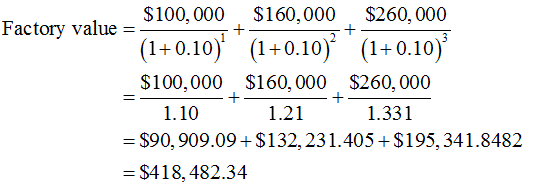# A factory costs \$320,000. You forecast that it will produce cash inflows if \$100,000 in a year, \$160,000 in year 2, and \$260,000 in year 3. The discount rate is 10%.what is the value if the factory?

Question
39 views

A factory costs \$320,000. You forecast that it will produce cash inflows if \$100,000 in a year, \$160,000 in year 2, and \$260,000 in year 3. The discount rate is 10%.

what is the value if the factory?

check_circle

Step 1

The value of factory can be determined with the help of below expression:Step 2

Factory value is calculated below:...

### Want to see the full answer?

See Solution

#### Want to see this answer and more?

Solutions are written by subject experts who are available 24/7. Questions are typically answered within 1 hour.*

See Solution
*Response times may vary by subject and question.
Tagged in